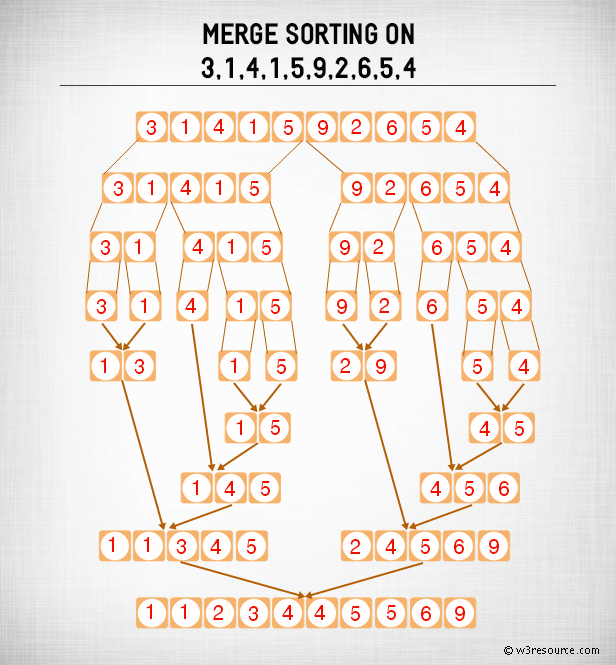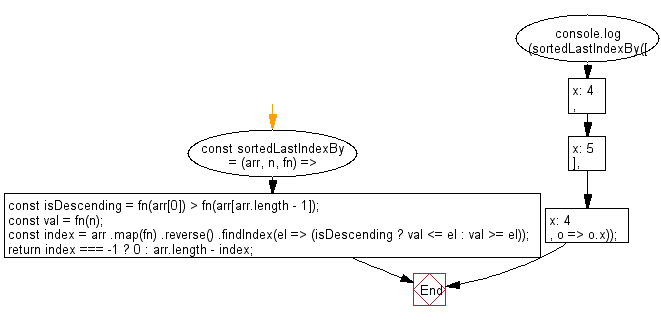﻿ PHP: Sort a list of elements using Merge sort - w3resource# PHP Searching and Sorting Algorithm: Merge sort

## PHP Searching and Sorting Algorithm: Exercise-17 with Solution

Write a PHP program to sort a list of elements using Merge sort.

According to Wikipedia "Merge sort (also commonly spelled mergesort) is an O (n log n) comparison-based sorting algorithm. Most implementations produce a stable sort, which means that the implementation preserves the input order of equal elements in the sorted output."

Pictorial presentation - Merge search algorithm :Sample Solution :

PHP Code :

``````<?php
function merge_sort(\$my_array){
if(count(\$my_array) == 1 ) return \$my_array;
\$mid = count(\$my_array) / 2;
\$left = array_slice(\$my_array, 0, \$mid);
\$right = array_slice(\$my_array, \$mid);
\$left = merge_sort(\$left);
\$right = merge_sort(\$right);
return merge(\$left, \$right);
}
function merge(\$left, \$right){
\$res = array();
while (count(\$left) > 0 && count(\$right) > 0){
if(\$left > \$right){
\$res[] = \$right;
\$right = array_slice(\$right , 1);
}else{
\$res[] = \$left;
\$left = array_slice(\$left, 1);
}
}
while (count(\$left) > 0){
\$res[] = \$left;
\$left = array_slice(\$left, 1);
}
while (count(\$right) > 0){
\$res[] = \$right;
\$right = array_slice(\$right, 1);
}
return \$res;
}
\$test_array = array(100, 54, 7, 2, 5, 4, 1);
echo "Original Array : ";
echo implode(', ',\$test_array );
echo "\nSorted Array :";
echo implode(', ',merge_sort(\$test_array))."\n";
?>
```
```

Sample Output:

```Original Array : 100, 54, 7, 2, 5, 4, 1
Sorted Array : 1, 2, 4, 5, 7, 54, 100
```

Flowchart :PHP Code Editor:

Have another way to solve this solution? Contribute your code (and comments) through Disqus.

What is the difficulty level of this exercise?

Test your Programming skills with w3resource's quiz.

﻿

## PHP: Tips of the Day

PHP: "date(): It is not safe to rely on the system's timezone settings..."

You probably need to put the timezone in a configuration line in your php.ini file. You should have a block like this in your php.ini file:

```[Date]
; Defines the default timezone used by the date functions
; http://php.net/date.timezone
date.timezone = America/New_York
```

If not, add it (replacing the timezone by yours). After configuring, make sure to restart httpd (service httpd restart).

Here is the list of supported timezones.

Ref : https://bit.ly/3kBe0Os